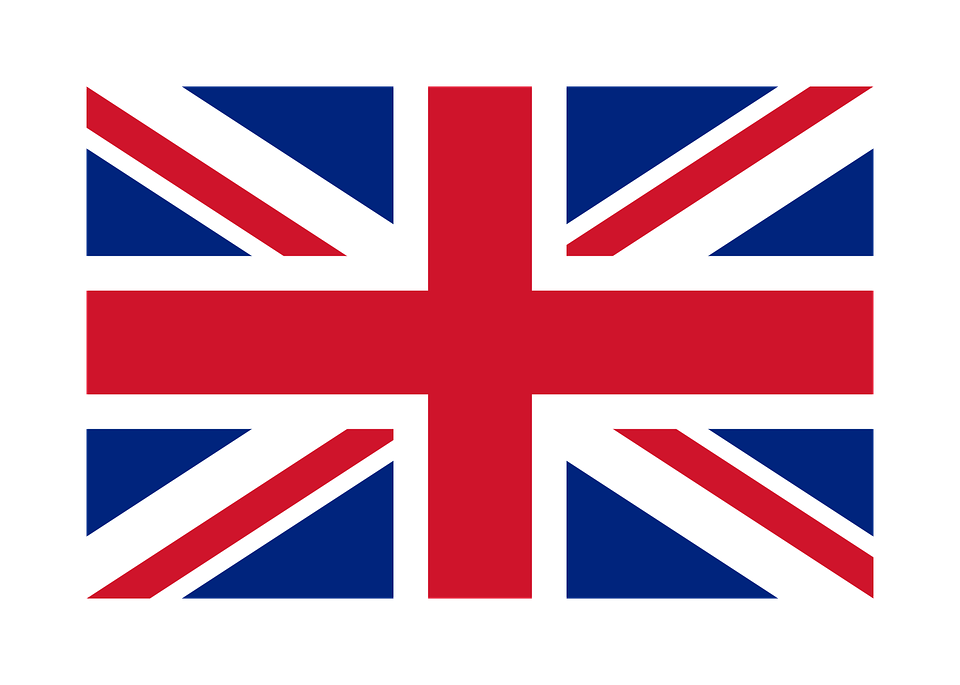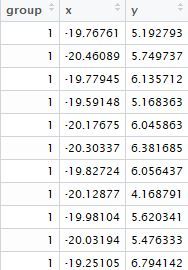# SIBER (SIAR package) in R：物种生态位重复度计算

(本文于 2016-5-27 00:10 首发于 “科学网”)
2018/02/01 updated 2017/10/05 updated 2017/3/24 updated 温馨提示：请确保您的 R软件（及R相关软件）安装的系统盘（e.g., C盘），这点十分重要！

SIBER: Stable Isotope Bayesian Ellipses in R

“SIBER” (SIAR package)这个SIAR套件的SIBER分析，主要是用来比较一个生态系统内不同种群之间的同位素生态位竞争的具体百分比值，等等。

Fits bivariate ellipses to stable isotope data using Bayesian inference with the aim being to describe and compare their isotopic niche.``````rm(list=ls())
setwd("E:/")
graphics.off()
# 我的数据集包含 3 列: group,x,y.
# x, y 表示 d13C 和 d15N 的值.
``````

``````library(siar)
attach(mydata)
# now loop through the data and calculate the ellipses.
ngroups<-length(unique(group))

#split the isotope data based on group.
spx<-split(x,group)
spy<-split(y,group)

#create some empty vectors for recording our metrics.
SEA<-numeric(ngroups)
SEAc<-numeric(ngroups)
TA<-numeric(ngroups)

# plot the raw data.
# dev.new() #maybe no need show the plot in a new window.
plot(x,y,col=group,type="p")
legend("topright",legend=as.character(paste("Group",unique(group))),pch=19,col=1:length(unique(group)))
``````

Now loop over each group and plot the small sample size corrected ellipse and the convex hull for each.

``````for(j in unique(group)){

#fit a standard ellipse to the data.
SE<-standard.ellipse(spx[[j]],spy[[j]],steps=1)

#extract the estimated SEA and SEAc from this object.
SEA[j]<-SE\$SEA
SEAc[j]<-SE\$SEAc

#plot the stand ellipse with d.f.=2(i.e.SEAc).
#these are plotted here as thick solid lines.
lines(SE\$xSEAc,SE\$ySEAc,col=j,lty=1,lwd=3)   ###

#also,for comparison we can fit and plot the convex hull.
#the convex hull is plotted as dotted thin lines.
# calculate the convex hull for the jth group's isotope values.
# held in the objects created using split()called spx and spy.
CH<-convexhull(spx[[j]],spy[[j]])

#extract the area of the convex hull from this object.
TA[j]<-CH\$TA

#plot the convex hull
lines(CH\$xcoords,CH\$ycoords,lwd=1,lty=3)     ###
}
``````

print the area metrics to screen for comparison.

``````#NB if you are working with real data rather than simulated then you wont be able to calculate the population SEA(pop.SEA).

#if you do this enough times or for enough groups you will easily see the bias in SEA as an estimate of pop.SEA as compared to SEAc which is unbiased.

#both measures are equally variable.

print(cbind(SEA,SEAc,TA))
``````

So for we have fitted the standard ellipses based on frequentist methods and calculated the relevant metrics(SEA and SEAc).

Now we turn our attention to producing a Bayesian estimate of the standard ellipse and its area SEA.B.

``````reps<-10^4  #循环计算次数.

# Generate the Bayesian estimates for the SEA for each group using the utility function siber.ellipses.

SEA.B<-siber.ellipses(x,y,group,R=reps)
summary(SEA.B)    #N.B. SEA.B数据集包含  10^4 行的内容.
``````

plot out some of the data and results.

Compare two ellipses for significant differences in SEA.

To test whether Group 1 SEA is smaller than Group 2…

You need to calculate the Proportion of G1 ellipses that are less than G2.

To calculate the overlap between two ellipses you can use the following code

One might then wish to calculate the proportion overlap; athough one then runs into a choice as to what the demoninator will be in the equation. You could for instance calculate

the proportion of A that overlaps with B,

the proporiton of B that overlaps with A,

or the proportion of A and B that overlap with each other.

You can also cacluate the overlap between two of the convex hulls, or indeed any polygon using the code that underlies the overlap() function.

And calculate the overlap using the function in spatstat package.

Q & A

For this case from siar package, data only need 3 columns as iso1,iso2,group.

<已有 次阅读>

① 本文仅代表作者个人观点，不代表任何其它立场，欢迎交流合作！

② 转载与分享请注明：本文源于 http://meiweiping.cn